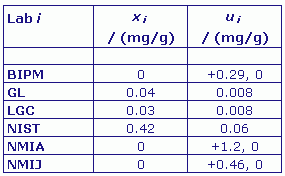Comparison
Comparison close
Results

MEASURAND : Mass fraction of Estradiol in the CCQM-K55.a samples

Degrees of equivalence : offset Di and expanded uncertainty (k = 2) Ui expressed in mg/g, and also in relative terms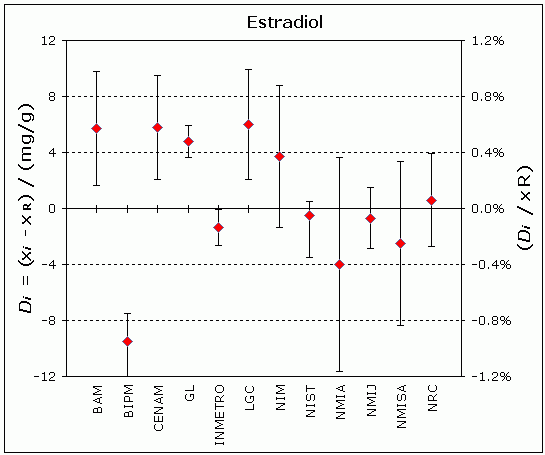xR = 984.3 mg/g
2 uR = 0.8 mg/g

MEASURAND : Mass fraction of Related Structure Impurities in the CCQM-K55.a samples

Degrees of equivalence : offset Di and expanded uncertainty (k = 2) Ui expressed in mg/g, and also in relative terms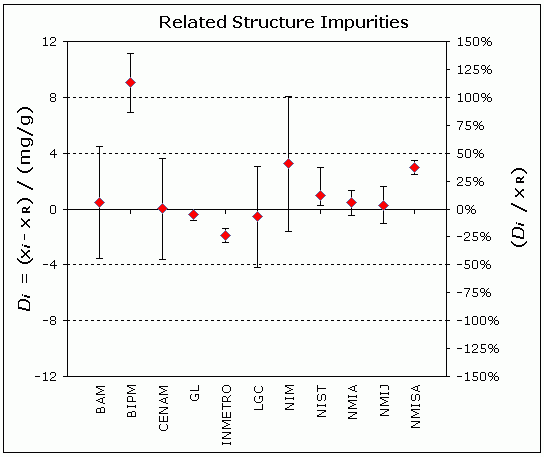xR = 8.65 mg/g
2 uR = 0.32 mg/g

MEASURAND : Mass fraction of Water in the CCQM-K55.a samples

Degrees of equivalence : offset Di and expanded uncertainty (k = 2) Ui expressed in mg/g, and also in relative terms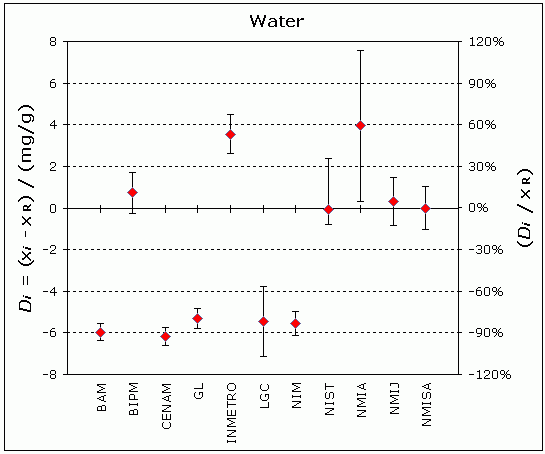xR = 6.75 mg/g
2 uR = 0.42 mg/g

MEASURAND : Mass fraction of Volatile Organics in the CCQM-K55.a samples

Degrees of equivalence : offset Di and expanded uncertainty (k = 2) Ui expressed in mg/g, and also in relative terms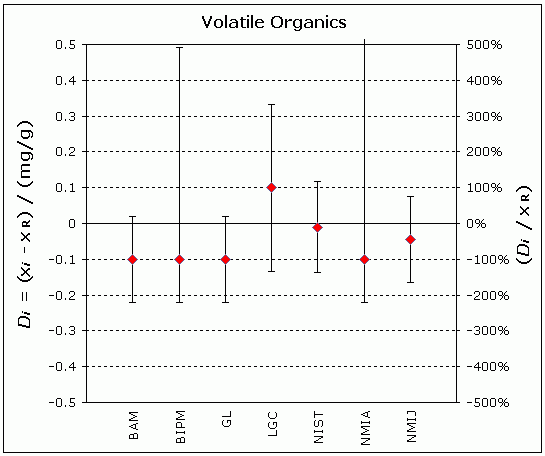xR = 0.10 mg/g
2 uR = 0.12 mg/g

MEASURAND : Mass fraction of Non-Volatile Content in the CCQM-K55.a samples

Degrees of equivalence : offset Di and expanded uncertainty (k = 2) Ui expressed in mg/g, and also in relative terms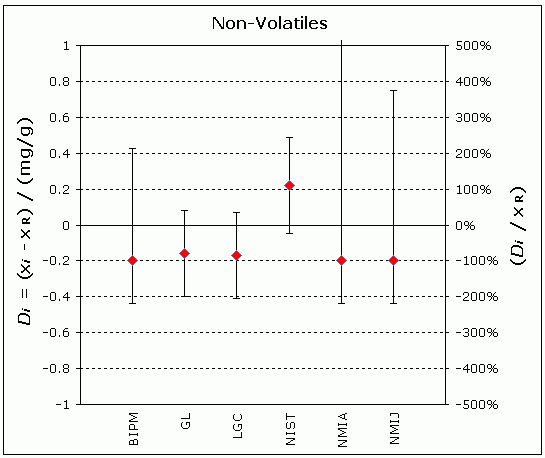xR = 0.20 mg/g
2 uR = 0.24 mg/g

Comparison
Comparison close
Results

MEASURAND : Mass fraction of Estradiol in the CCQM-K55.a samples

Degrees of equivalence : offset Di and expanded uncertainty (k = 2) Ui expressed in mg/g
xR = 984.3 mg/g and 2 uR = 0.8 mg/g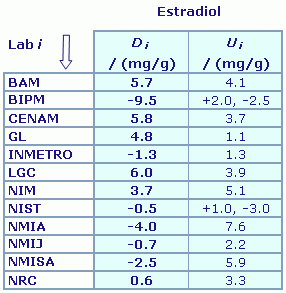Results are presented under A4 printable format in Summary Results (PDF file).

MEASURAND : Mass fraction of Related Structure Impurities in the CCQM-K55.a samples

Degrees of equivalence : offset Di and expanded uncertainty (k = 2) Ui expressed in mg/g
xR = 8.65 mg/g and 2 uR = 0.32 mg/g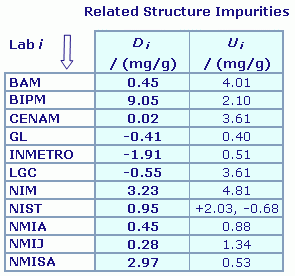Results are presented under A4 printable format in Summary Results (PDF file).

MEASURAND : Mass fraction of Water in the CCQM-K55.a samples

Degrees of equivalence : offset Di and expanded uncertainty (k = 2) Ui expressed in mg/g
xR = 6.75 mg/g and 2 uR = 0.42 mg/g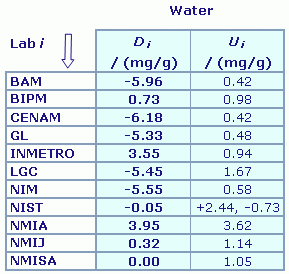Results are presented under A4 printable format in Summary Results (PDF file).

MEASURAND : Mass fraction of Volatile Organics in the CCQM-K55.a samples

Degrees of equivalence : offset Di and expanded uncertainty (k = 2) Ui expressed in mg/g
xR = 0.10 mg/g and 2 uR = 0.12 mg/g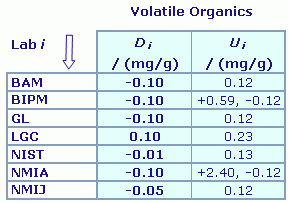Results are presented under A4 printable format in Summary Results (PDF file).

MEASURAND : Mass fraction of Non-Volatile Content in the CCQM-K55.a samples

Degrees of equivalence : offset Di and expanded uncertainty (k = 2) Ui expressed in mg/g
xR = 0.20 mg/g and 2 uR = 0.24 mg/g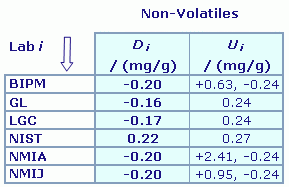Results are presented under A4 printable format in Summary Results (PDF file).

Comparison
Comparison close
CC comparison
 Metrology area, Sub-field Chemistry and Biology, Organics Description Purity assessment of high purity organic materials: 17beta-Estradiol Time of measurements 2008 - 2009 Status Approved for equivalence
 Final Reports of the comparisons Measurand Mass fraction of 17beta-EstradiolNominal value > 970 mg/g Transfer device Dedicated samples
 Comparison type Key Comparison Consultative Committee CCQM (Consultative Committee for Amount of Substance) Conducted by CCQM (Consultative Committee for Amount of Substance) Comments Results published on 22 August 2012 Purity assessment of high purity organic materials: 17beta-Estradiol Pilot institute BIPM Bureau International des Poids et Mesures BIPM - International Organization Contact person Steven Westwood +33 (0) 1 45 07 70 57
First Name Last Name
wwww@ww.www +356719836 Institute 1 Institute 1 Khmelnitskiy
Pilot laboratory
BIPM

Bureau International des Poids et Mesures, BIPM - International Organization, N/A

 BAM Bundesanstalt für Materialforschung und -prüfung, Germany, EURAMET
 CENAM Centro Nacional de Metrologia, Mexico, SIM
 GL Government Laboratory, Hong Kong, China, APMP
 INMETRO Instituto Nacional de Metrologia, Qualidade e Tecnologia, Brazil, SIM
 LGC Laboratory of the Government Chemist, United Kingdom, EURAMET
 NIM National Institute of Metrology, China, APMP
 NIST National Institute of Standards and Technology, United States, SIM
 NMIA National Measurement Institute, Australia, Australia, APMP
 NMIJ AIST National Metrology Institute of Japan, Japan, APMP
 NMISA National Metrology Institute of South Africa, South Africa, AFRIMETS
 NRC National Research Council, Canada, SIM
Comparison
Comparison close
Results

MEASURAND : Mass fraction of Estradiol in the CCQM-K55.a samples

 The key comparison reference value for the mass fraction of estradiol, xR, was calculated by subtraction from the limit value of 1000 mg/g of the summation of the individual estimates for each contributing class of impurities present in CCQM-K55.a samples, as explained in page 21 of the Final Report. Its standard uncertainty, uR, is computed by combination in quadrature of the uncertainties associated with each contributing impurity estimate and also uncertainties in the inter-unit homogeneity of water and related structure impurity content.xR = 984.3 mg/g, uR = 0.41 mg/g

 The degree of equivalence of laboratory i with respect to the key comparison reference value is given by a pair of terms: Di = (xi - xR) and Ui, its expanded uncertainty (k = 2), both expressed in mg/g, with Ui = 2(ui2 + uR2)1/2.

 No pair-wise degrees of equivalence are computed for this comparison.

MEASURAND : Mass fraction of Related Structure Impurities in the CCQM-K55.a samples

 Key comparison reference values (xR) and associated standard uncertainty (uR) were assigned for the mass fraction of each orthogonal impurity class -  related substances, water, volatile organics and non-volatiles - quantified by the participants in CCQM-K55.a who used a mass balance approach to assign the estradiol content. They are consensus values derived from the individual data supplied by each participant. The assignment method varied by impurity class and each is separately described on pages 17 to page 20 of the Final Report. For Total Related Structure Impurities, xR = 8.65 mg/g, uR = 0.16 mg/g

 The degree of equivalence of laboratory i with respect to the key comparison reference value is given by a pair of terms: Di = (xi - xR) and Ui, its expanded uncertainty (k = 2), both expressed in mg/g, with Ui = 2(ui2 + uR2)1/2.

 No pair-wise degrees of equivalence are computed for this comparison.

MEASURAND : Mass fraction of Water in the CCQM-K55.a samples

 Key comparison reference values (xR) and associated standard uncertainty (uR) were assigned for the mass fraction of each orthogonal impurity class - related substances, water, volatile organics and non-volatiles - quantified by the participants in CCQM-K55.a who used a mass balance approach to assign the estradiol content. They are consensus values derived from the individual data supplied by each participant. The assignment method varied by impurity class and each is separately described on pages 17 to page 20 of the Final Report. For Water, xR = 6.75 mg/g, uR = 0.21 mg/g

 The degree of equivalence of laboratory i with respect to the key comparison reference value is given by a pair of terms: Di = (xi - xR) and Ui, its expanded uncertainty (k = 2), both expressed in mg/g, with Ui = 2(ui2 + uR2)1/2.

 No pair-wise degrees of equivalence are computed for this comparison.

MEASURAND : Mass fraction of Volatile Organics in the CCQM-K55.a samples

 Key comparison reference values (xR) and associated standard uncertainty (uR) were assigned for the mass fraction of each orthogonal impurity class - related substances, water, volatile organics and non-volatiles - quantified by the participants in CCQM-K55.a who used a mass balance approach to assign the estradiol content. They are consensus values derived from the individual data supplied by each participant. The assignment method varied by impurity class and each is separately described on pages 17 to page 20 of the Final Report. For Volatile Organics, xR = 0.10 mg/g, uR = 0.06 mg/g

 The degree of equivalence of laboratory i with respect to the key comparison reference value is given by a pair of terms: Di = (xi - xR) and Ui, its expanded uncertainty (k = 2), both expressed in mg/g, with Ui = 2(ui2 + uR2)1/2.

 No pair-wise degrees of equivalence are computed for this comparison.

MEASURAND : Mass fraction of Non-Volatile Content in the CCQM-K55.a samples

 Key comparison reference values (xR) and associated standard uncertainty (uR) were assigned for the mass fraction of each orthogonal impurity class - related substances, water, volatile organics and non-volatiles - quantified by the participants in CCQM-K55.a who used a mass balance approach to assign the estradiol content. They are consensus values derived from the individual data supplied by each participant. The assignment method varied by impurity class and each is separately described on pages 17 to page 20 of the Final Report. For Non-Volatile Content, xR = 0.20 mg/g, uR = 0.12 mg/g

 The degree of equivalence of laboratory i with respect to the key comparison reference value is given by a pair of terms: Di = (xi - xR) and Ui, its expanded uncertainty (k = 2), both expressed in mg/g, with Ui = 2(ui2 + uR2)1/2.

 No pair-wise degrees of equivalence are computed for this comparison.
Comparison
Comparison close
Results

MEASURAND : Mass fraction of Estradiol in the CCQM-K55.a samples

xi : result of measurement carried out by laboratory i
ui : combined standard uncertainty of xi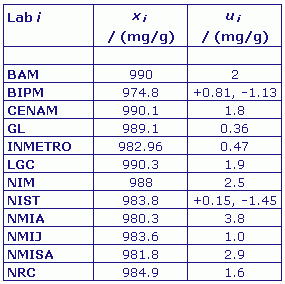MEASURAND : Mass fraction of Related Structure Impurities in the CCQM-K55.a samples

xi : result of measurement carried out by laboratory i
ui : combined standard uncertainty of xi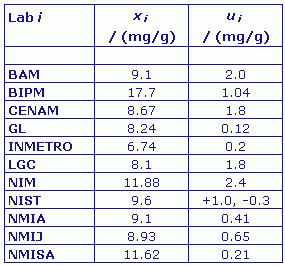MEASURAND : Mass fraction of Water in the CCQM-K55.a samples

xi : result of measurement carried out by laboratory i
ui : combined standard uncertainty of xi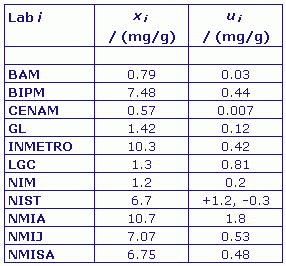MEASURAND : Mass fraction of Volatile Organics in the CCQM-K55.a samples

xi : result of measurement carried out by laboratory i
ui : combined standard uncertainty of xi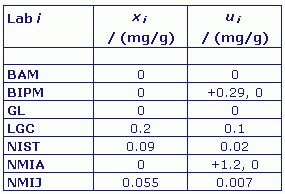MEASURAND : Mass fraction of Non-Volatile Content in the CCQM-K55.a samples

xi : result of measurement carried out by laboratory i
ui : combined standard uncertainty of xi